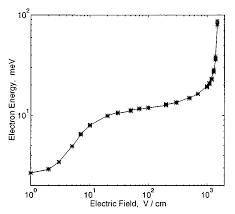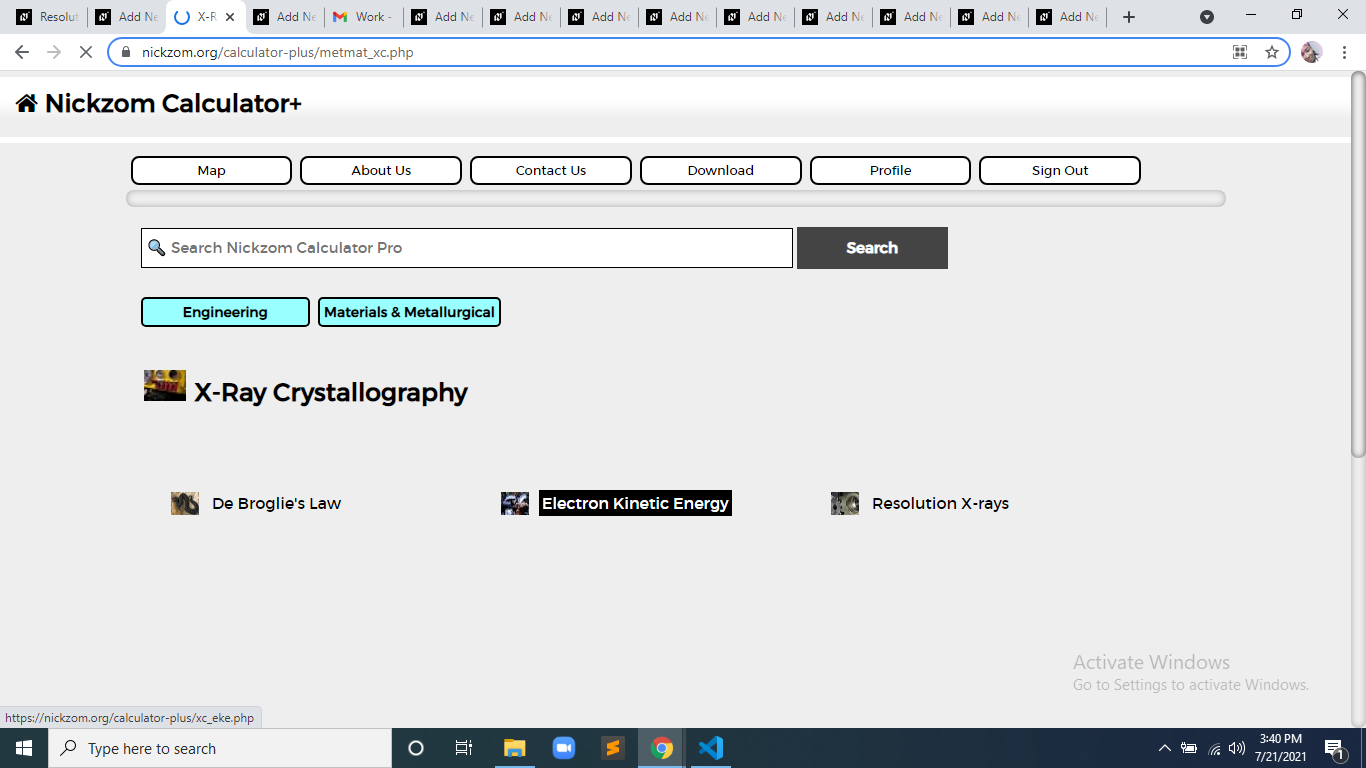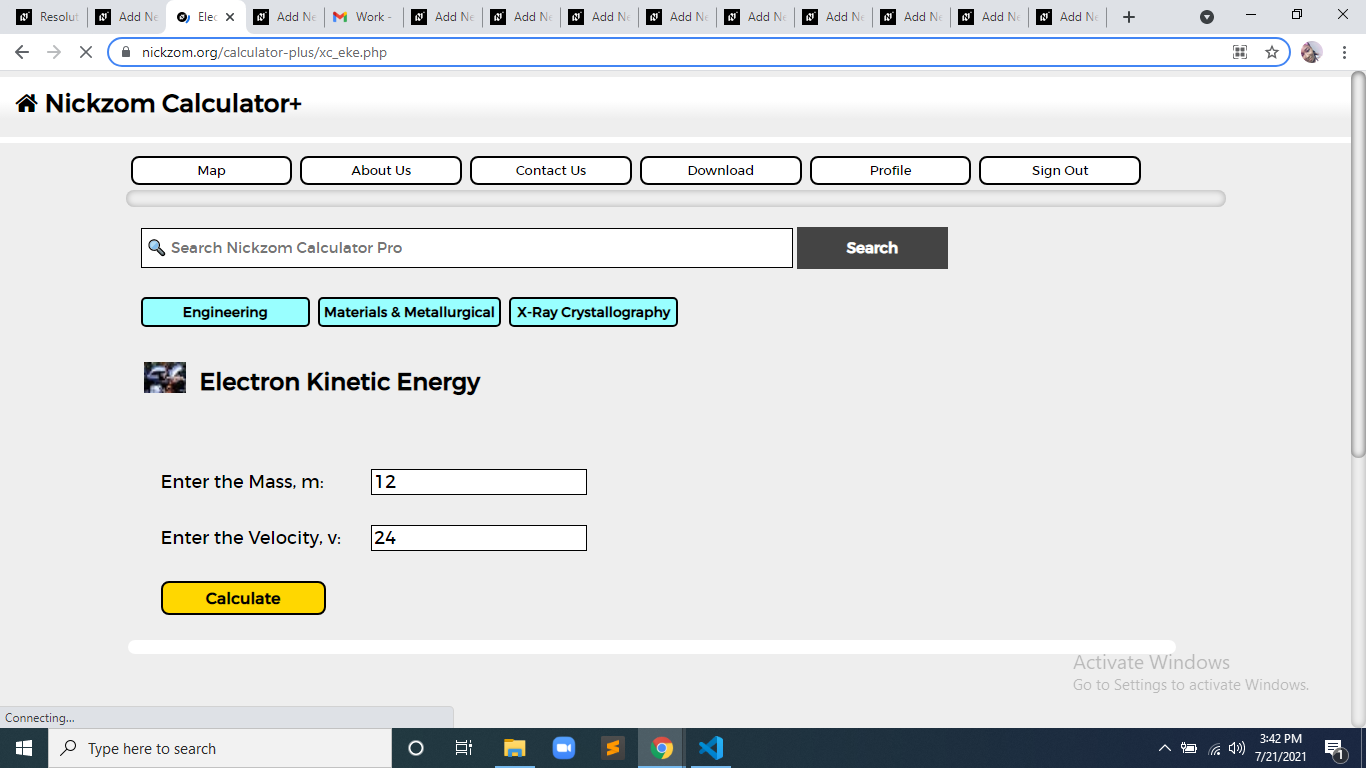# How to Calculate and Solve for Electron Kinetic Energy | X-Ray CrystallographyThe image above represents electron kinetic energy.

To compute for electron kinetic energy, two essential parameters are needed and these parameters are Mass (m) and Velocity (v).

The formula for calculating electron kinetic energy:

eV = mv²/2

Where:

eV = Electron Kinetic Energy
m = Mass
v = Velocity

Let’s solve an example;
Find the electron kinetic energy when the mass is 12 and velocity is 24.

This implies that;

m = Mass = 12
v = Velocity = 24

eV = mv²/2
eV = (12)(24)²/2
eV = (12)(576)/2
eV = (6912)/2
eV = 3456

Therefore, the electron kinetic energy is 3456 J.

Calculating the Mass when the Electron Kinetic Energy and the Velocity is Given.

m = eV x 2 / v2

Where;

m = Mass
eV = Electron Kinetic Energy
v = Velocity

Let’s solve an example;
Find the mass when the electron kinetic energy is 12 and the velocity is 4.

This implies that;

eV = Electron Kinetic Energy = 12
v = Velocity = 4

m = eV x 2 / v2
m = 12 x 2 / 42
m = 24 / 16
m = 1.5

Therefore, the mass is 1.5.

Calculating the Velocity when the Electron Kinetic Energy and the Mass is Given.

v = √(eV x 2 / m)

Where;

v = Velocity
eV = Electron Kinetic Energy
m = Mass

Let’s solve an example;
Find the velocity when the electron kinetic energy is 10 and the mass is 8.

This implies that;

eV = Electron Kinetic Energy = 10
m = Mass = 8

v = √(eV x 2 / m)
v = √(10 x 2 / 8)
v = √(20 / 8)
v = √2.5
v = 1.58

Therefore, the velocity is 1.58.

Nickzom Calculator – The Calculator Encyclopedia is capable of calculating the electron kinetic energy.

To get the answer and workings of the electron kinetic energy using the Nickzom Calculator – The Calculator Encyclopedia. First, you need to obtain the app.

You can get this app via any of these means:

To get access to the professional version via web, you need to register and subscribe for NGN 2,000 per annum to have utter access to all functionalities.
You can also try the demo version via https://www.nickzom.org/calculator

Apple (Paid) – https://itunes.apple.com/us/app/nickzom-calculator/id1331162702?mt=8
Once, you have obtained the calculator encyclopedia app, proceed to the Calculator Map, then click on Materials and Metallurgical under Engineering.Now, Click on X-ray Crystallography under Materials and MetallurgicalNow, Click on Electron Kinetic Energy under X-ray CrystallographyThe screenshot below displays the page or activity to enter your values, to get the answer for the electron kinetic energy according to the respective parameters which is the Mass (m) and Velocity (v).Now, enter the value appropriately and accordingly for the parameter as required by the Mass (m) is 12 and Velocity (v) is 24.Finally, Click on CalculateAs you can see from the screenshot above, Nickzom Calculator– The Calculator Encyclopedia solves for the electron kinetic energy and presents the formula, workings and steps too.